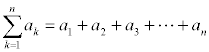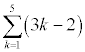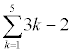# 7.1 - Sequences and Summation Notation

A sequence is a function whose domain is the natural numbers. Instead of using the f(x) notation, however, a sequence is listed using the an notation. There are infinite sequences whose domain is the set of all positive integers, and there are finite sequences whose domain is the set of the first n positive integers.

When you define a sequence, you must write the general term (nth term or an). There are sometimes more than one sequence that is possible if just the first few terms are given.

## Defining a Sequence

There are two common ways to define a sequence by specifying the general term.

### General Term, an

The first is to use a form that only depends on the number of the term, n. To find the first five terms when you know the general term, simply substitute the values 1, 2, 3, 4, and 5 into the general form for n and simplify.

Consider the sequence defined by the general term an = 3n-2

The first five terms are found by plugging in 1, 2, 3, 4, and 5 for n.

1. 3(1) - 2 = 1
2. 3(2) - 2 = 4
3. 3(3) - 2 = 7
4. 3(4) - 2 = 10
5. 3(5) - 2 = 13

Therefore, the first five terms of the sequence are 1, 4, 7, 10, 13

Now consider the sequence defined by the general term an = 1 / n.

The first five terms are 1/1, 1/2, 1/3, 1/4, and 1/5.

### Recursive Definition

The second way is to recursively define a sequence. A recursive definition uses the current and/or previous terms to define the next term. You can think of ak+1 being the next term, ak being the current term, and ak-1 being the previous term.

Consider the sequence where a1 = 5 and ak+1 = 2 ak - 1.

You can read that last part as "the next term is one less than twice the current term"

The first five terms are:

1. 5 (by definition),
2. 2(5) - 1 = 9 (twice the first term of 5 minus 1),
3. 2(9) - 1 = 17 (twice the second term of 9 minus 1),
4. 2(17) - 1 = 33 (twice the third term of 17 minus 1),
5. 2(33) - 1 = 62 (twice the fourth term of 33 minus 1)

Now consider the sequence defined by a1 = 2, a2 = 1, and ak+2 = 3ak - ak+1

You can read that last part as "the next term is 3 times the last term minus the current term"

The first five terms are:

1. 2 (by definition),
2. 1 (by definition),
3. 3(2) - 1 = 5 (3 times first term minus second term), Note that when k = 1, the sequence gets written as a1+2 = 3a1 - a1+1 which becomes a3 = 3a1 - a2. Since a1 = 2 and a2 = 1, this is where the 3(2) - 1 = 5 comes from.
4. 3(1) - 5 = -2 (3 times second term minus third term),
5. 3(5) - (-2) = 17 (3 times third term minus fourth term)

## Fibonacci Sequence

One famous example of a recursively defined sequence is the Fibonacci Sequence. The first two terms of the Fibonacci Sequence are 1 by definition. Every term after that is the sum of the two preceding terms.

The Fibonacci Sequence is 1, 1, 2, 3, 5, 8, 13, 21, 34, 55, 89, ... an+1 = an + an-1.

Fibonacci sequences occur frequently in nature. For example, take a leaf on a stem of many plants (like cherry, elm, or pear trees). Count the number of leaves until you reach one directly in line with the one you selected. The total number of leaves (not including the first one) is usually a Fibonacci number. If the left and right handed spirals on a pine cone, sunflower seed heads, or pineapples are counted, the two numbers are often consecutive Fibonacci numbers.

## Factorials - !

The symbol for factorial is ! (an explanation point). The factorial of a positive integer is the product of all positive integers less than or equal to that number. Zero factorial is a special case and 0! = 1 by defintion.

1! = 1
2! = 2*1! = 2*1 = 2
3! = 3*2! = 3*2*1 = 6
4! = 4*3! = 4*3*2*1 = 24
5! = 5*4! = 5*4*3*2*1 = 120

n! = n(n-1)! = n*(n-1)*(n-2)*...3*2*1

Notice that there is also a recursive definition in there. Any number factorial is that number times the factorial of one less than that number.

On the TI-82 and TI-83 calculators, the factorial key can be found under Math, Probability, menu choice 4.

### Simplifying ratios of factorials

Consider 8!/5!. One way to work the problem would be to fully expand the 8! and fully expand the 5!.

```8!   8 * 7 * 6 * 5 * 4 * 3 * 2 * 1
-- = ----------------------------- = 8 * 7 * 6 = 336
5!               5 * 4 * 3 * 2 * 1```

Then you notice that there are common factors of 5, 4, 3, 2, and 1 in both the numerator and denominator that divide out. This leaves you with 8*7*6 in the numerator for an answer of 336.

Another way to work the problem, however is to use the recursive nature of factorials. Since any number factorial is that number times the factorial of one less than that number, 8! = 8 * 7!, but 7! = 7 * 6!, and 6! = 6 * 5!. This means that 8! = 8 * 7 * 6 * 5!. So, 8! / 5! Is 8*7*6*5!/5! = 8*7*6 = 336.

The point is that there is no need to multiply the entire thing out when you're just going to be dividing a bunch of it out anyway.

Here are some steps to simplifying the ratio of two factorials.

1. Find the smaller factorial and write it down. It won't change, and it will be the part that is completely divided out.
2. Take the larger factorial and start expanding it by subtracting one until the smaller number (that you've already written down) is reached.
3. When you reach the smaller number, write it as a factorial and divide out the two equal factorials.

Here's an example with a little bit more complicated ratio. Since 2n-1 is less than 2n+1, copy the 2n-1 factorial down on the bottom. Now, take 2n+1 times one less than 2n+1. One less then 2n+1 is 2n+1-1=2n. Take that times one less than 2n, which is 2n-1. Since that 2n-1 is the same amount on the bottom, call the 2n-1 factorial and then divide it out, leaving the product of 2n and 2n+1 as the answer.

```(2n+1)!   (2n+1)*(2n)*(2n-1)!
------- = ------------------- = 2n(2n+1)
(2n-1)!               (2n-1)!```

## Sigma / Summation Notation

Summation is something that is done quite often in mathematics, and there is a symbol that means summation. That symbol is the capital Greek letter sigma, and so the notation is sometimes called Sigma Notation instead of Summation Notation.The k is called the index of summation. k=1 is the lower limit of the summation and k=n (although the k is only written once) is the upper limit of the summation.

What the summation notation means is to evaluate the argument of the summation for every value of the index between the lower limit and upper limit (inclusively) and then add the results together.

### Examples:Substitute each value of k between 1 and 5 into the expression 3k-2 and then add the results together.

[3(1)-2] + [3(2)-2] + [3(3)-2] + [3(4)-2] + [3(5)-2] = 1 + 4 + 7 + 10 + 13 = 35[ 3(1) + 3(2) + 3(3) + 3(4) + 3(5) ] - 2 = [ 3 + 6 + 9 + 12 + 15 ] - 2 = 45 - 2 = 43

Although these look very similar, the answers are different. The second example is thrown in there to warn you about notation. Multiplication has a higher order of operations than addition or subtraction, so no group symbols are needed around the 3k. But since subtraction has the same precedence as addition, the subtraction of 2 does not go inside the summation. In other words, be sure to include parentheses around a sum or difference if you want the summation to apply to more than just the first term.

## Properties of Summation

The following properties of summation apply no matter what the lower and upper limits are for the index. For simplicity sake, I will not write the k=1 and n, but know that your index of summation is k in the following examples.

### You can factor a constant out of a sum.

∑cak = c∑ak

Notice the ak has a subscript of k while the c doesn't. This means that the c is a constant and the a is function of k. The sum of a constant times a function is the constant times the sum of the function.

### The sum of a sum is the sum of the sums

Ooh, that just sounds good. The summation symbol can be distributed over addition.

∑(ak + bk) = ∑ak + ∑bk

### The sum of a difference is the difference of the sums

The summation symbol can be distributed over subtraction.

∑(ak - bk) = ∑ak - ∑bk

Aren't those just beautiful sounding? Those should certainly be placed on a note card to help you remember them.